### Introduction

The Random Forest algorithm is one of the most flexible, powerful and widely-used algorithms for classification and regression, built as an ensemble of Decision Trees.

If you aren’t familiar with these – no worries, we’ll cover all of these concepts.

In this in-depth hands-on guide, we’ll build an intuition on how decision trees work, how ensembling boosts individual classifiers and regressors, what random forests are and build a random forest classifier and regressor using Python and Scikit-Learn, through an end-to-end mini-project, and answer a research question.

Consider that you’re are currently part of a research group that is analysing data about women. The group has collected 100 data records and wants to be able to organize those initial records by dividing the women into categories: being or not pregnant, and living in rural or urban areas. The researchers want to understand how many women would be in each category.

There is a computational structure that does exactly that, it is the tree structure. By using a tree structure, you will be able to represent the different divisions for each category.

### Decision Trees

How do you populate the nodes of a tree? This is where decision trees come into focus.

First, we can divide the records by pregnancy, after that, we can divide them by living in urban or rural areas. Notice, that we could do this in a different order, dividing initially by what area the women live and after by their pregnancy status. From this, we can see that the tree has an inherent hierarchy. Besides organizing information, a tree organizes information in a hierarchical manner – the order that the information appears matters and leads to different trees as a result.

Below, is an example of the tree that has been described: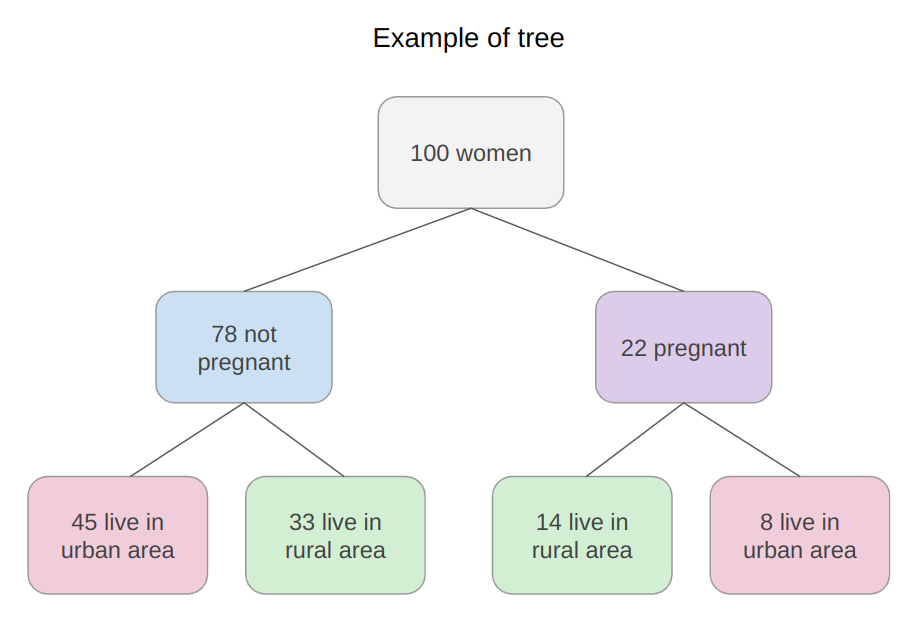In the tree image, there are 7 squares, the one on top that accounts for the total of 100 womem, this top square is connected with two squares below, that divide the women based on their number of 78 not pregnant and 22 pregnant, and from both previous squares there are four squares; two connected to each square above that divide the women based on their area, for the not pregnant, 45 live in an urban area, 33 in a rural area and for the pregnant, 14 live in a rural area and 8 in an urban area. Just by looking a the tree, it is easy to understand those divisions and see how each “layer” is derived from previous ones, those layers are the tree levels, the levels describe the depth of the tree: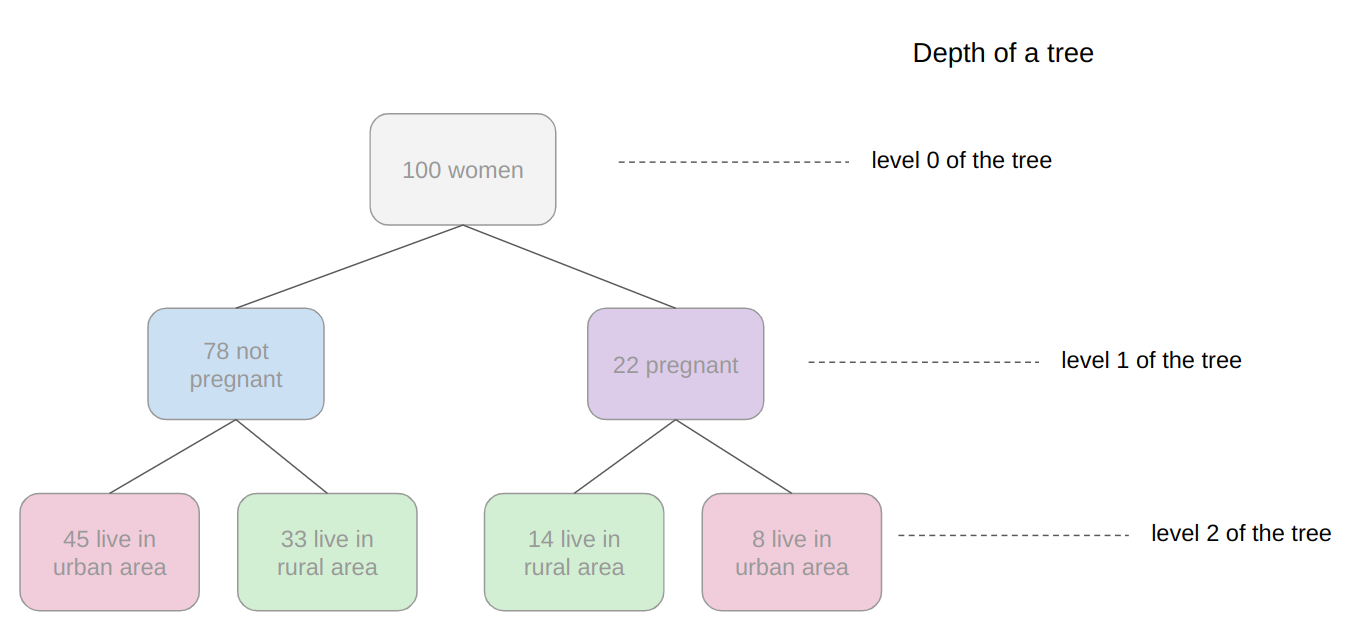Observe in the image above that the first tree level is level 0 where there is only one square, followed by level 1 where there are two squares, and level 2 where there are four squares. This is a depth 2 tree.

In level 0 is the square that originates the tree, the first one, called root node, this root has two child nodes in level 1, that are parent nodes to the four nodes in level 2. See that the “squares” we have been mentioning so far, are actually called nodes; and that each previous node is a parent to the following nodes, that are its children. The child nodes of each level that have the same parent are called siblings, as can be seen in the next image: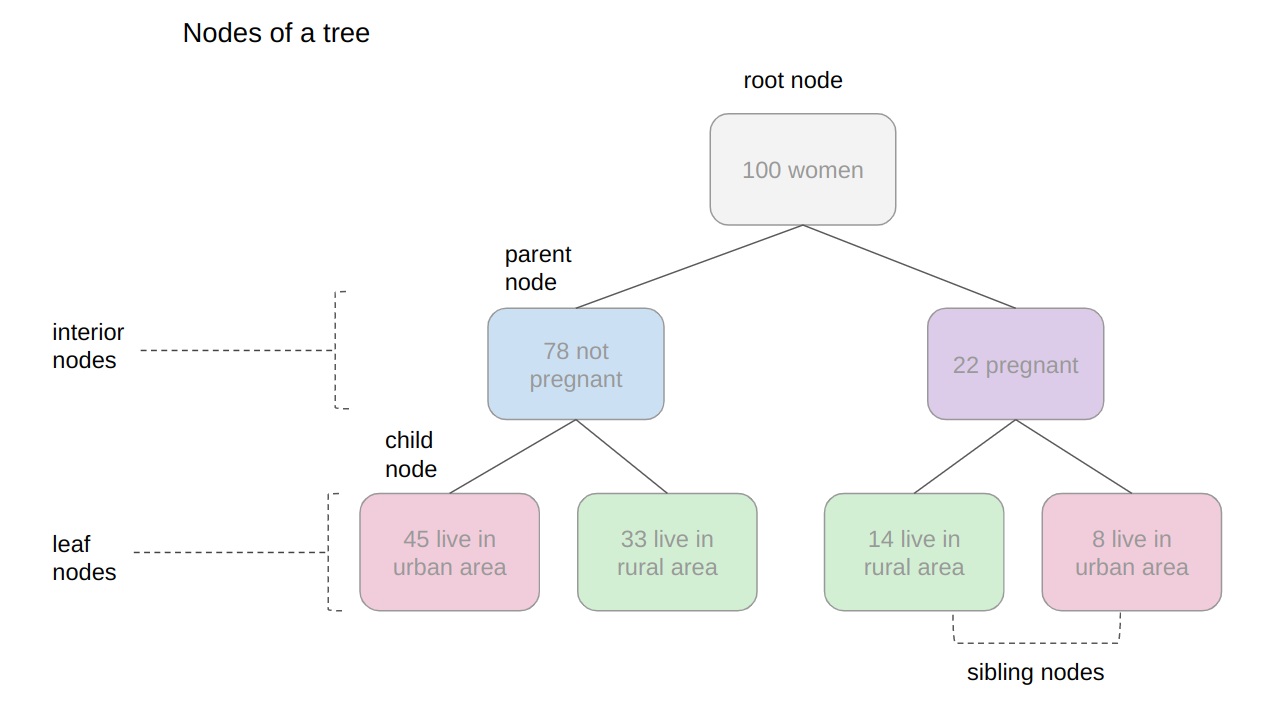In the previous image, we also display the level 1 as being the interior nodes, once they are between the root and the last nodes, which are the leaf nodes. The leaf nodes are the last part of a tree, if we were to say from the 100 initial women, how many are pregnant and living in rural areas, we could do this by looking at the leaves. So the number at the leaves would answer the first research question.

If there were new records of women, and the tree that was previously used to categorize them, was now used to decide if a woman could or couldn’t be part of the research, would it still function? The tree would use the same criteria, and a woman would be ellegible to participate if pregnant and living in a rural area.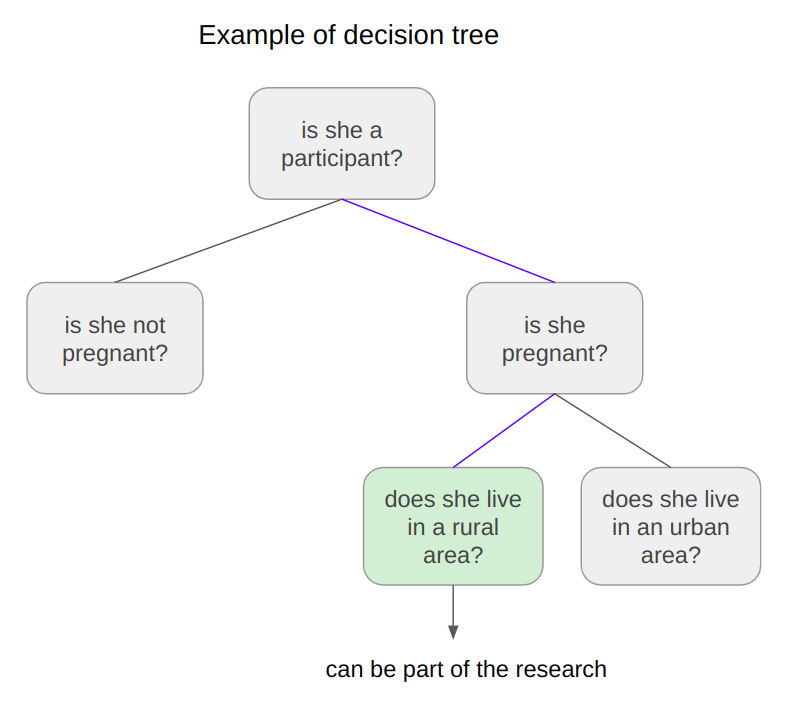By looking at the image above, we can see that the answers to the questions of each tree node – “is she a participant?”, “is she pregnant?”, “does she live in a rural area?”- are yes, yes, and yes, so it seems that the tree can ideed lead to a decision, in this case, that the woman could take part in the research.

This is the essense of decision trees in, done in a manual manner. Using Machine Learning, we can construct a model that constructs this tree automatically for us, in such a way to maximize the accuracy of the final decisions.Note: there are several types of trees in Computer Science, such as binary trees, general trees, AVL trees, splay trees, red black trees, b-trees, etc. Here, we are focusing on giving a general idea of what is a decision tree. If it depends on the answer of a yes or no question for each node and thus each node has at most two children, when sorted so that the “smaller” nodes are on the left, this classifies decision trees as binary trees.

In the previous examples, observe how the tree could either classify new data as participant or non participant, or the questions could also be changed to – “how many are participants?”, “how many are pregnant?”, “how many live in a rural area?”- leading us to find the quantity of pregnant participants that live in a rural area.

When the data is classified, this means the tree is performing a classification task, and when the quantity of data is found, the tree is performing a regression task. This means that the decision tree can be used for both tasks – classification and regression.

Now that we understand what a decision tree is, how can it be used, and what nomenclature is used to describe it, we can wonder about its limitations.

### Understanding Random Forests

What happens to the decision if some participant lives on the division between urban and rural areas? Would the tree add this record to rural or urban? It seems hard to fit this data into the structure we currently have, since it’s fairly clear cut.

Also, what if a woman that lives on a boat participates in the research, would it be considered rural or urban? In the same way as the previous case, it is a challeging data point to classify considering the available options in the tree.

By thinking a bit more about the decision tree example, we are able to see it can correctly classify new data considering it already follows a pattern the tree already has – but when there are records that differ from the initial data that defined the tree, the tree structure is too rigid, making the records not classifiable.

This means that the decision tree can be strict and limited in its possibilities. An ideal decision tree would be more flexible and able to accomodate more nuanced unseen data.Solution: Just as “two pairs of eyes see better than one”, two models typically come up with a more accurate answer than one. Accounting for the diversity in knowledge representations (encoded in the tree structure), the rigidity of the sligtly different structures between multiple similar trees aren’t as limiting anymore, since the shortcomings of one tree can be “made up for” by another. By combining many trees together, we get a forest.

Regarding the answer to the initial question, we already know that it will be encoded in the tree leaves – but what changes when we have many trees instead of one?

If the trees are combined for a classification, the result will be defined by the majority of answers, this is called majority voting; and in the case of a regression, the number given by each tree in the forest will be averaged.

#### Ensemble Learning and Model Ensembles

This method is known as ensemble learning. When empliying ensemble learning, you can mix any algorithms together, as long as you can ensure that the output can be parsed and combined with other outputs (either manually, or using existing libraries). Typically, you ensemble multiple models of the same type together, such as multiple decision trees, but you’re not limited to just join same-model type ensembles.

Ensembling is a practically guaranteed way to generalize better to a problem, and to squeeze out a slight performance boost. In some cases, ensembling models yields a significant increase in predictive power, and sometimes, just slight. This depends on the dataset you’re training and evaluating on, as well as the models themselves.

Joining decision trees together yields significant performance boosts compred to individual trees. This approach was popularized in the research and applied machine learning communities, and was so common that the ensemble of decision trees was colloquially named a forest, and the common type of forest that was being created (a forest of decision trees on a random subset of features) popularized the name random forests.

Given wide-scale usage, libraries like Scikit-Learn have implemented wrappers for RandomForestRegressors and RandomForestClassifiers, built on top of their own decision tree implementations, to allow researchers to avoid building their own ensembles.

Let’s dive into random forests!

### How the Random Forest Algorithm Works?

The following are the basic steps involved when executing the random forest algorithm:

1. Pick a number of random records, it can be any number, such as 4, 20, 76, 150, or even 2.000 from the dataset (called N records). The number will depend on the width of the dataset, the wider, the larger N can be. This is where the random part in the algorithm’s name comes from!
2. Build a decision tree based on those N random records;
3. According to the number of trees defined for the algorithm, or the number of trees in the forest, repeat steps 1 and 2. This generates more trees from sets of random data records;
4. After step 3, comes the final step, which is predicting the results:
• In case of classification: each tree in the forest will predict the category to which the new record belongs. After that, the new record is assigned to the category that wins the majority vote.
• In case of regression: each tree in the forest predicts a value for the new record, and the final prediction value will be calculated by taking an average of all the values predicted by all the trees in the forest.

Each tree fit on a random subset of features will necessarily have no knowledge of some other features, which is rectified by ensembling, while keeping the computational cost lower.Advice: Since Random Forest use Decision Trees as a base, it is very helpful to understand how Decision Trees work and have some practice with them individually to build an intuition on their structure. When composing random forests, you’ll be setting values such as the maximum depth of a tree, the minimum number of sampels required to be at a leaf node, the criteria to bet determine internal splits, etc. to help the ensemble better fit a dataset, and generalize to new points. In practice, you’ll typically be using Random Forests, Gradient Boosting or Extreme Gradient Boosting or other tree-based methodologies, so having a good grasp on the hyperparameters of a single decision tree will help with building a strong intuition for tuning ensembles.

With an intuition on how trees work, and an understanding of Random Forests – the only thing left is to practice building, training and tuning them on data!

### Building and Training Random Forest Models with Scikit-Learn

There was a reason for the examples used so far involve pregnancy, living area and women.

In 2020, researchers from Bangladesh noticed that the mortality among pregnant women was still very high, specially considering ones that live in rural areas. Because of that, they used an IOT monitoring system to analyse the risk of maternal health. The IOT system collected data from different hospitals, community clinics, and maternal health cares from the rural areas of Bangladesh.

The collected data was then organized in a comma-separated-value (csv) file and uploaded to UCI’s machine learning repository.Note: you can read more about the research work in the Review and Analysis of Risk Factor of Maternal Health in Remote Area Using the Internet of Things (IoT)” paper.

This is the data that we will use to practice and try to understand if a pregnant woman has a low, medium or high risk of mortality.### Using Random Forest for Classification

Since we want to know if woman has a low, medium or high risk of mortality, this means we will perform a classification with three classes. When a problem has more than two classes, it is called a multiclass problem, as opposed to a binary problem (where you choose between two classes, typically 0 and 1).

In this first example, we will implement a multiclass classification model with a Random Forest classifier and Python’s Scikit-Learn.

We will follow the usual machine learning steps to solve this problem, which are loading libraries, reading the data, looking at summary statistics and creating data visualizations to better understand it. Then, preprocessing and splitting the data followed by generating, training and evaluating a model.

##### Importing Libraries

We’ll be using Pandas to read the data, Seaborn and Matplotlib to visualize it, and NumPy for the great utility methods:

import pandas as pd
import numpy as np
import seaborn as sns
import matplotlib.pyplot as plt

##### Importing the Dataset

The following code imports the dataset and loads it into a python DataFrame:

dataset = pd.read_csv("../../datasets/random-forest/maternal_health_risk.csv")


To look at the first five lines of the data, we execute the head() command:

dataset.head()


This outputs:

 Age SystolicBP DiastolicBP BS BodyTemp HeartRate RiskLevel
0 25 130 80 15.0 98.0 86 high risk
1 35 140 90 13.0 98.0 70 high risk
2 29 90 70 8.0 100.0 80 high risk
3 30 140 85 7.0 98.0 70 high risk
4 35 120 60 6.1 98.0 76 low risk


Here we can see all the attributes collected during the research.

• Age: ages in years.
• SystolicBP: upper value of Blood Pressure in mmHg, a significant attribute during pregnancy.
• DiastolicBP: lower value of Blood Pressure in mmHg, another significant attribute during pregnancy.
• BS: blood glucose levels in terms of a molar concentration, mmol/L.
• HeartRate: resting heart rate in beats per minute.
• RiskLevel: risk level during pregnancy.
• BodyTemp: the body temperature.

Now that we understand more about what is being measured, we can look at the types of data with info():

dataset.info()


This results in:

<class 'pandas.core.frame.DataFrame'>
RangeIndex: 1014 entries, 0 to 1013
Data columns (total 7 columns): # Column Non-Null Count Dtype --- ------ -------------- ----- 0 Age 1014 non-null int64 1 SystolicBP 1014 non-null int64 2 DiastolicBP 1014 non-null int64 3 BS 1014 non-null float64 4 BodyTemp 1014 non-null float64 5 HeartRate 1014 non-null int64 6 RiskLevel 1014 non-null object dtypes: float64(2), int64(4), object(1)
memory usage: 55.6+ KB


From looking at the RangeIndex line, we can see that there are 1014 records, and the column Non-Null Count informs that the data doesn’t have any missing values. This means, we won’t need to make any treatment for missing data!

In the Dtype column, we can see the type of each variable. Currently, float64 columns such BS and BodyTemp have numerical values that may vary in any range, such as 15.0, 15.51, 15.76, 17.28, making them numerically continuous (you can always add a 0 to a floating point number, ad infinitem). On the other hand, variables such as Age, SystolicBP, DiastolicBP, and HeartRate are of the type int64, this means that the numbers only change by the unit, such as 11, 12, 13, 14 – we won’t have a heart rate of 77.78, it is either 77 or 78 – those are numerically discrete values. And we also have RiskLevel with a object type, this usually indicates that the variable is a text, and we will probably need to transform it into a number. Since the risk level grows from low to high, there is an implied order in the categories, this indicates it is a categorically ordinal variable.Note: it is important to look at the type of each data, and see if it makes sense according to its context. For instance, it doesn’t make sense to have half of a heart rate unit, so this means the interger type is adequate for a discrete value. When that doesn’t happen, you can change the type of the data with Pandas’ astype() propety – df['column_name'].astype('type').

After looking at data types, we can use describe() to take a peak at some descriptive statistics, such as the mean values of each column, the standard deviation, quantiles, minimum and maximum data values:

dataset.describe().T # T transposes the table


The above code displays:

 count mean std min 25% 50% 75% max
Age 1014.0 29.871795 13.474386 10.0 19.0 26.0 39.0 70.0
SystolicBP 1014.0 113.198225 18.403913 70.0 100.0 120.0 120.0 160.0
DiastolicBP 1014.0 76.460552 13.885796 49.0 65.0 80.0 90.0 100.0
BS 1014.0 8.725986 3.293532 6.0 6.9 7.5 8.0 19.0
BodyTemp 1014.0 98.665089 1.371384 98.0 98.0 98.0 98.0 103.0
HeartRate 1014.0 74.301775 8.088702 7.0 70.0 76.0 80.0 90.0
RiskLevel 1014.0 0.867850 0.807353 0.0 0.0 1.0 2.0 2.0


Notice that for most columns, the mean values are far from the standard deviation (std) – this indicates that the data doesn’t necessarily follow a well behaved statistical distribution. If it did, it would’ve helped the model when predicting the risk. What can be done here is to preprocess the data to make it more representative as if it were data from the whole world population, or more normalized. But, an advantage when using Random Forest models for classification, is that the inherent tree structure can deal well with data that has not been normalized, once it divides it by the value in each tree level for each variable.

Also, because we are using trees and that the resulting class will be obtained by voting, we aren’t inherently comparing between different values, only between same types of values, so adjusting the features to the same scale isn’t necessary in this case. This means that the Random Forest classification model is scale invariant, and you don’t need to perform feature scaling.

In this case, the step in data preprocessing we can take is to transform the categorical RiskLevel column into a numerical one.

##### Visualizing the Data

Before transforming RiskLevel, let’s also quickly visualize the data by looking at the combinations of points for each pair of features with a Scatterplot and how the points are distributed by visualizing the histogram curve. To do that, we will use Seaborn’s pairplot() which combines both plots. It generates both plots for each feature combination and displays the points color coded according to their risk level with the hue property:

g = sns.pairplot(dataset, hue='RiskLevel')
g.fig.suptitle("Scatterplot and histogram of pairs of variables color coded by risk level", fontsize = 14, # defining the size of the title y=1.05); # y = definig title y position (height)


The above code generates: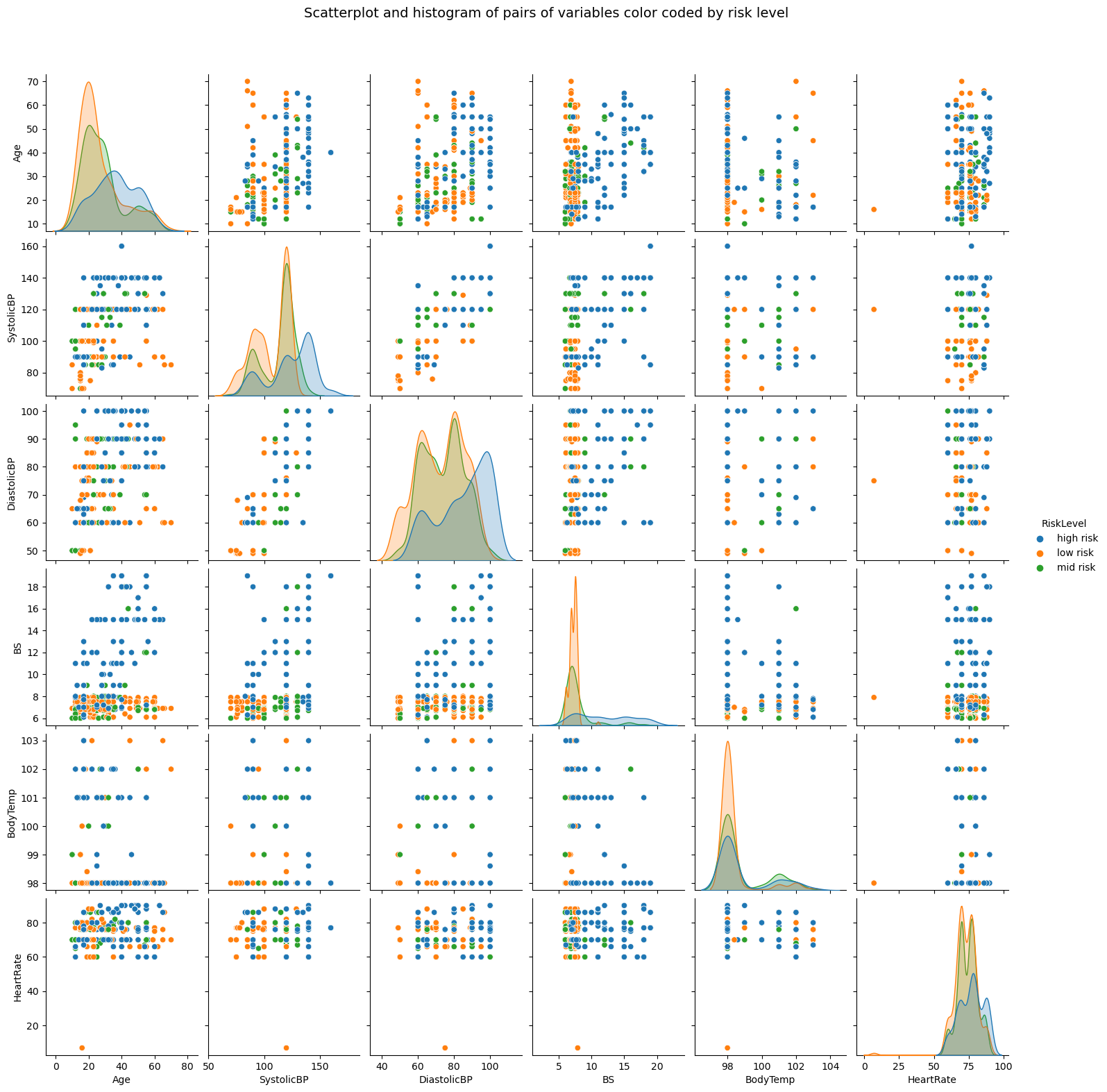When looking at the plot, the ideal situation would be to have a clear separation between curves and dots. As we can see, the three types of risk classes are mostly mixed up, since trees internally draw lines when delimiting the spaces between points, we can hypothesize that more trees in the forest might be able to limit more spaces and better classify the points.Advice: if you want to visualize how the trees work internally, you can create a Decision Boundary Plot. This process is covered in our Byte-sized tutorial on “Plot Decision Boundaries Using Python and Scikit-Learn”.

With the basic exploratory data analysis done, we can preprocess the RiskLevel column.

##### Data Preprocessing for Classification

To be sure there are only three classes of RiskLevel in our data, and that no other values have been added erroneously, we can use unique() to display the column’s unique values:

dataset['RiskLevel'].unique()


This outputs:

array(['high risk', 'low risk', 'mid risk'], dtype=object)


The classes are checked, now the next step is to transform each value into a number. Since there is an order between classifications, we can use the values 0, 1 and 2 to signify low, medium and high risks. There are many ways to change the column values, following Python’s simple is better that complex motto, we will use the .replace() method, and simply replace them with their integer representations:

dataset['RiskLevel'] = dataset['RiskLevel'].replace('low risk', 0).replace('mid risk', 1).replace('high risk', 2)


After replacing the values, we can divide the data into what will be used for training the model, the features or X, and what we want to predict, the labels or y:

y = dataset['RiskLevel']
X = dataset.drop(['RiskLevel'], axis=1)


Once the X and y sets are ready, we can use Scikit-Learn’s train_test_split() method to further divide them into the train and test sets:

from sklearn.model_selection import train_test_split SEED = 42
X_train, X_test, y_train, y_test = train_test_split(X, y, test_size=0.2, random_state=SEED)Advice: remember to use a random state seed if you want to make the result reproducible. We’ve used a random state seed so you can reproduce the same results as from the guide.

Here, we are using 20% of the data for testing and 80% for training.

##### Training a RandomForestClassifier

Scikit-Learn implemented ensembles under the sklearn.ensemble module. An ensemble of decision trees used for classification, in which a majority vote is taken is implemented as the RandomForestClassifier.

Having the train and test sets, we can import the RandomForestClassifier class and create the model. To start, let’s create a forest with three trees, by setting n_estimators parameter as 3, and with each tree having three levels, by setting max_depthto 2:

from sklearn.ensemble import RandomForestClassifier rfc = RandomForestClassifier(n_estimators=3, max_depth=2, random_state=SEED)Note: The default value for n_estimators is 100. This boosts the predictive power and generalization of the ensemble, but we’re creating a smaller one to make it easier to visualize and inspect it. With just 3 trees – we can visualize and inspect them manually to further build our intuition of both the individual trees, and their co-dependence. The same applies for max_depth, which is None, meaning the trees can get deeper and deeper to fit the data as required.

To fit the model around the data – we call the fit() method, pasing in the training features and labels:

# Fit RandomForestClassifier
rfc.fit(X_train, y_train)
# Predict the test set labels
y_pred = rfc.predict(X_test)


We can now compare the predicted labels against the real labels to evaluate how well the model did! Before evaluating the model, let’s take a look into the ensemble.

To look a little deeper into the model, we can visualize each of the trees and how they are dividing the data. This can be done by using the tree module built into Scikit-Learn, and then looping through each of the estimators in the ensemble:

# Import tree module
from sklearn import tree features = X.columns.values # The name of each column
classes = ['0', '1', '2'] # The name of each class
# You can also use low, medium and high risks in the same order instead
# classes = ['low risk', 'medium risk', 'high risk'] for estimator in rfc.estimators_: print(estimator) plt.figure(figsize=(12,6)) tree.plot_tree(estimator, feature_names=features, class_names=classes, fontsize=8, filled=True, rounded=True) plt.show()


The above code displays the tree plots: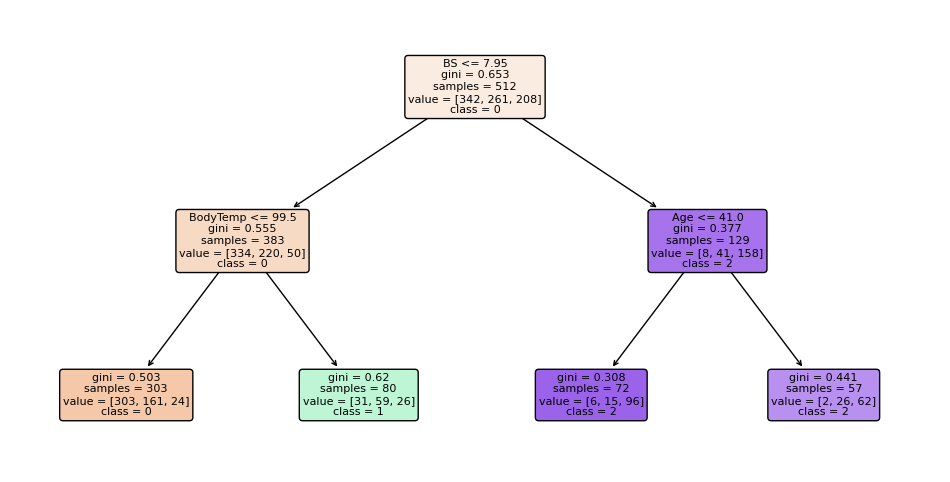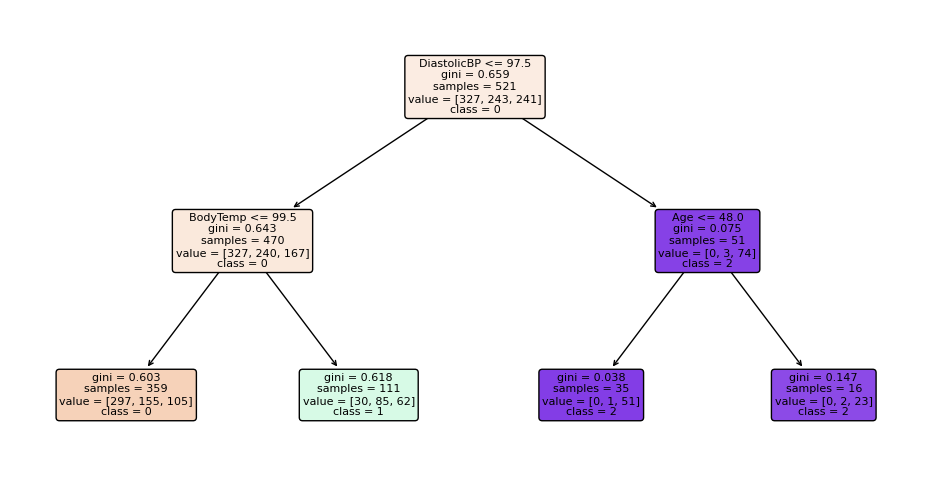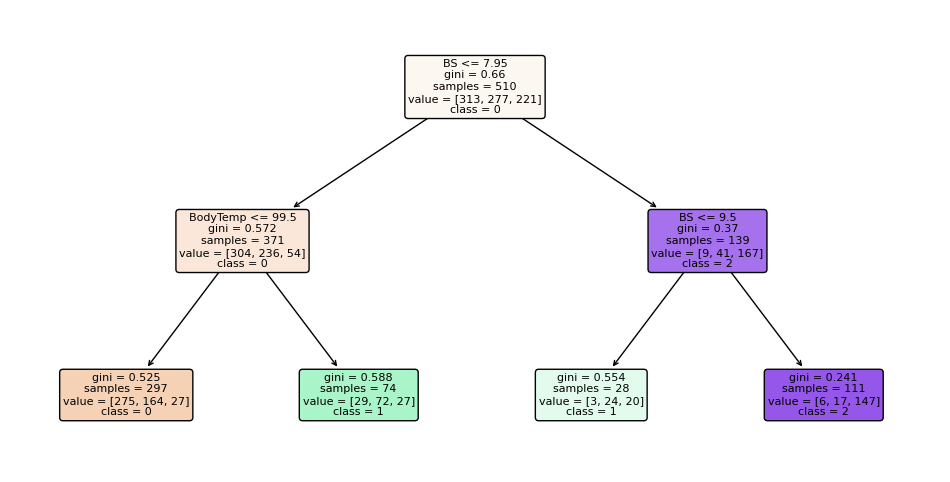Advice: If you want to learn more about plotting trees, to not digress here, check out the Byte-sized tutorial on “Plotting Decision Trees Using Python and Scikit-Learn”!

Notice how the three trees are different. The first one starts with the BS feature, the second with DiastolicBP, and the third with BS again. Although the third looks at a different number of samples. On the right branch, the first two trees also decide using Age at the leaf level, while the third tree ends with BS feature. With just three estimators, it’s clear how scaling up gives a rich, diverse representation of the knowledge that can be successfully ensembled into a highly-accurate model.

The more trees in the forest, the more diverse the model can be. There’s a point of diminishing returns, though, as with many trees fit on a random subset of features, there will be a fair bit of similar trees that don’t offer much diversity in the ensemble, and which will start to have too much voting power and skew the ensemble to be overfit on the training dataset, hurting generalization to the validation set.

There was a hypotesis made earlier about having more trees, and how it could improve the model results. Let’s take a look at the results, generate a new model and see if the hipothesis holds!

##### Evaluating the RandomForestClassifier

Scikit-Learn makes it easy to create baselines by providing a DummyClassifier, which outputs predictions without using the input features (totally random outputs). If your model is better than the DummyClassifier, some learning is happening! To maximize the learning – you can test out various hyperparameters automatically by using a RandomizedSearchCV or GridSearchCV. Besides having a baseline, you can evaluate your model’s performance from the lens of several metrics.

Some traditional classification metrics that can be used to evaluate the algorithm are precision, recall, f1-score, accuracy, and confusion matrix. Here is a brief explanation on each of them:

1. Precision: when our aim is to understand what correct prediction values were considered correct by our classifier. Precision will divide those true positive values by the samples that were predicted as positives;

$$precision = \frac{\text{true positives}}{\text{true positives} + \text{false positives}}$$

1. Recall: commonly calculated along with precision to understand how many of the true positives were identified by our classifier. The recall is calculated by dividing the true positives by anything that should have been predicted as positive.

$$recall = \frac{\text{true positives}}{\text{true positives} + \text{false negatives}}$$

1. F1 score: is the balanced or harmonic mean of precision and recall. The lowest value is 0 and the highest is 1. When f1-score is equal to 1, it means all classes were correctly predicted – this is a very hard score to obtain with real data (exceptions almost always exist).

$$\text{f1-score} = 2* \frac{\text{precision} * \text{recall}}{\text{precision} + \text{recall}}$$

1. Confusion Matrix: when we need to know how much samples we got right or wrong for each class. The values that were correct and correctly predicted are called true positives, the ones that were predicted as positives but weren’t positives are called false positives. The same nomenclature of true negatives and false negatives is used for negative values;

2. Accuracy: describes how many predictions our classifier got right. The lowest accuracy value is 0 and the highest is 1. That value is usually multiplied by 100 to obtain a percentage:

$$accuracy = \frac{\text{number of correct predictions}}{\text{total number of predictions}}$$Note: It’s practically impossible to obtain a 100% accuracy on any real data that you’d want to apply machine learning to. If you see a 100% accuracy classifier, or even a near-100% result – be skeptical, and perform evaluation. A common cause for these issues are data leakage (leaking part of the training test into a test set, directly or indirectly). There’s no consesnsus on what “a good accuracy is”, primarily because it depends on your data – sometimes, a 70% accuracy will be high! Sometimes, that’ll be a really low accuracy. Generally speaking, over 70% is sufficient for many models, but this is on the domain researcher to determine.

You can execute the following script to import the necessary libraries and look at the results:

from sklearn.metrics import classification_report, confusion_matrix cm = confusion_matrix(y_test, y_pred)
sns.heatmap(cm, annot=True, fmt='d').set_title('Maternal risks confusion matrix (0 = low risk, 1 = medium risk, 2 = high risk)') print(classification_report(y_test,y_pred))


The output will look something like this:

 precision recall f1-score support 0 0.53 0.89 0.66 80 1 0.57 0.17 0.26 76 2 0.74 0.72 0.73 47 accuracy 0.58 203 macro avg 0.61 0.59 0.55 203
weighted avg 0.59 0.58 0.53 203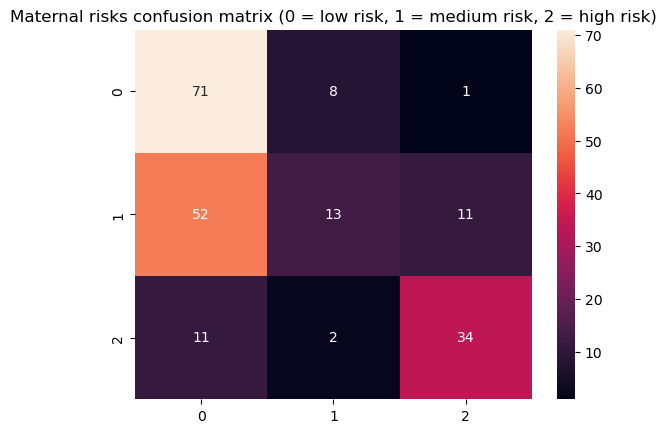In the classification report, observe that the recall is high, 0.89 for class 0, both precision and recall are high for class 2, 0.74, 0.72 – and for class 1, they are low, specially the recall of 0.17 and a precision of 0.57. The relationship between the recall and precision for all three classes individually is captured in the F1 score, which is the harmonic mean between recall and precision – the model is doing okay for class 0, fairly bad for class 1 and decent for class 2.

The model is having a very hard time when identifying the medium risk cases.

The accuracy achieved by our random forest classifier with only 3 trees is of 0.58 (58%) – this means it is getting the a bit more than half of the results right. This is a low accuracy, and perhaps could be improved by adding more trees.

By looking at the confusion matrix, we can see that most of the mistakes are when classifying 52 records of medium risk as low risk, what gives further insight to the low recall of class 1. It’s biased towards classifying medium-risk patients as low-risk patients.

Another thing that can be checked to generate even more insight is what features are most taken into consideration by the classifier when predicting. This is an important step to take for explainable machine learning systems, and helps identify and mitigate bias in models.

To see that, we can access the feature_importances_ property of the classifier. This will give us a list of percentages, so we can also access the feature_names_in_ property to get the name of each feature, organize them in a dataframe, sort them from highest to lowest, and plot the result:

# Organizing feature names and importances in a DataFrame
features_df = pd.DataFrame({'features': rfc.feature_names_in_, 'importances': rfc.feature_importances_ }) # Sorting data from highest to lowest
features_df_sorted = features_df.sort_values(by='importances', ascending=False) # Barplot of the result without borders and axis lines
g = sns.barplot(data=features_df_sorted, x='importances', y ='features', palette="rocket")
sns.despine(bottom = True, left = True)
g.set_title('Feature importances')
g.set(xlabel=None)
g.set(ylabel=None)
g.set(xticks=[])
for value in g.containers: g.bar_label(value, padding=2)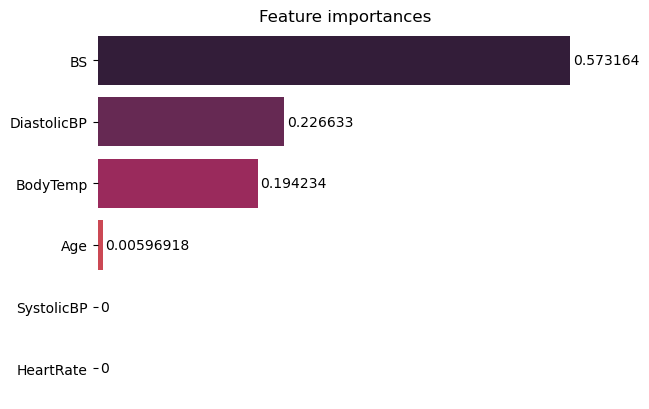Notice how the classifier is mostly considering the blood sugar, then a little of the diastolic pressure, body temperature and just a little of age to make a decision, this might also have to do with the low recall on class 1, maybe the medium risk data has to do with features that are not being taken into much consideration by the model. You can try to play around more with feature importances to investigate this, and see if changes on the model affect the features being used, also if there’s a significant relationship between some of the features and the predicted classes.

It is finally time to generate a new model with more trees to see how it affects the results. Let’s create the rfc_ forest with 900 trees, 8 levels and the same seed. Will the results improve?

rfc_ = RandomForestClassifier(n_estimators=900, max_depth=7, random_state=SEED)
rfc_.fit(X_train, y_train)
y_pred = rfc_.predict(X_test)


Calculating and displaying the metrics:

cm_ = confusion_matrix(y_test, y_pred)
sns.heatmap(cm_, annot=True, fmt='d').set_title('Maternal risks confusion matrix (0 = low risk, 1 = medium risk, 2 = high risk) for 900 trees with 8 levels') print(classification_report(y_test,y_pred))


This outputs:

 precision recall f1-score support 0 0.68 0.86 0.76 80 1 0.75 0.58 0.65 76 2 0.90 0.81 0.85 47 accuracy 0.74 203 macro avg 0.78 0.75 0.75 203
weighted avg 0.76 0.74 0.74 203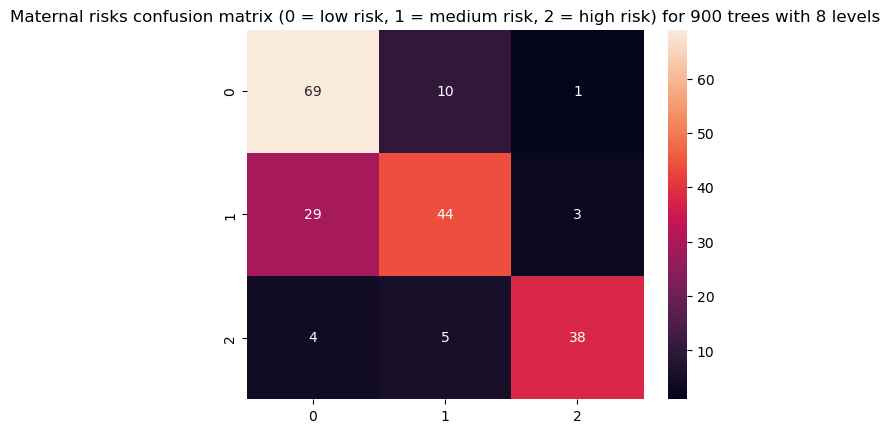This shows how adding more trees, and more specialized trees (higher levels), has improved our metrics. We still have a low recall for class 1, but the accuracy is now of 74%. The F1-score when classyfing high risk cases is of 0.85, which means high risk cases are now more easily identified when compared to 0.73 in the previous model!

In a day-to-day project, it might be more important to identify high risk cases, for instance with a metric similar to precision, which is also known as sensitivity in statistics. Try tweaking some of the model parameters and observe the results.

Up to now, we have obtained an overall understanding of how Random Forest can be used for classifying data – in the next section, we can use the same dataset in a different way to see how the same model predicts values with regression.

### Using Random Forests for Regression

In this section we will study how a Random Forest algorithm can be used to solve regression problems using Scikit-Learn. The steps followed to implement this algorithm are almost identical to the steps performed for classification, besides the type of model, and type of predicted data – that will now be continuous vales – there is only one difference in the data preparation.

Since regression is done for numerical values – let’s pick a numerical value from the dataset. We’ve seen that blood sugar was important in the classification, so it should be predictable based on other features (since if it correlates with some feature, that feature also correlates with it).

Following what we have done for classification, let’s first import the libraries and the same dataset. If you have already done this for the classification model, you can skip this part and go directly to preparing data for training.

##### Importing Libraries and Data
import pandas as pd
import numpy as np
import maplotlib.pyplot as plt
import seaborn as sns dataset = pd.read_csv("../../datasets/random-forest/maternal_health_risk.csv")

##### Data Preprocessing for Regression

This is a regression task, so instead of predicting classes, we can predict one of the numerical columns of the dataset. In this example, the BS column will be predicted. This means the y data will contain blood sugar data, and X data will contain all of the features besides blood sugar. After separating the X and y data, we can split the train and test sets:

from sklearn.model_selection import train_test_split SEED = 42 y = dataset['BS']
X = dataset.drop(['BS'], axis=1) # You can either include risk level or drop it here X_train, X_test, y_train, y_test = train_test_split(X, y, test_size=0.2, random_state=SEED)

##### Training a RandomForestRegressor

Now that we have scaled our dataset, it is time to train our algorithm to solve this regression problem, to change it up a bit – we’ll create a model with 20 trees in the forest and each one with 4 levels. To do it, you can execute the following code:

from sklearn.ensemble import RandomForestRegressor rfr = RandomForestRegressor(n_estimators=20, # 20 trees max_depth=3, # 4 levels random_state=SEED) rfr.fit(X_train, y_train)
y_pred = rfr.predict(X_test)


You can find details for all of the parameters of RandomForestRegressor in the official documentation.

Since plotting and looking at 20 trees would require some time and dedication, we can plot just the first one to have a look at how it is different from the classification tree:

from sklearn import tree features = X.columns
# Obtain just the first tree
first_tree = rfr.estimators_ plt.figure(figsize=(15,6))
tree.plot_tree(first_tree, feature_names=features, fontsize=8, filled=True, rounded=True);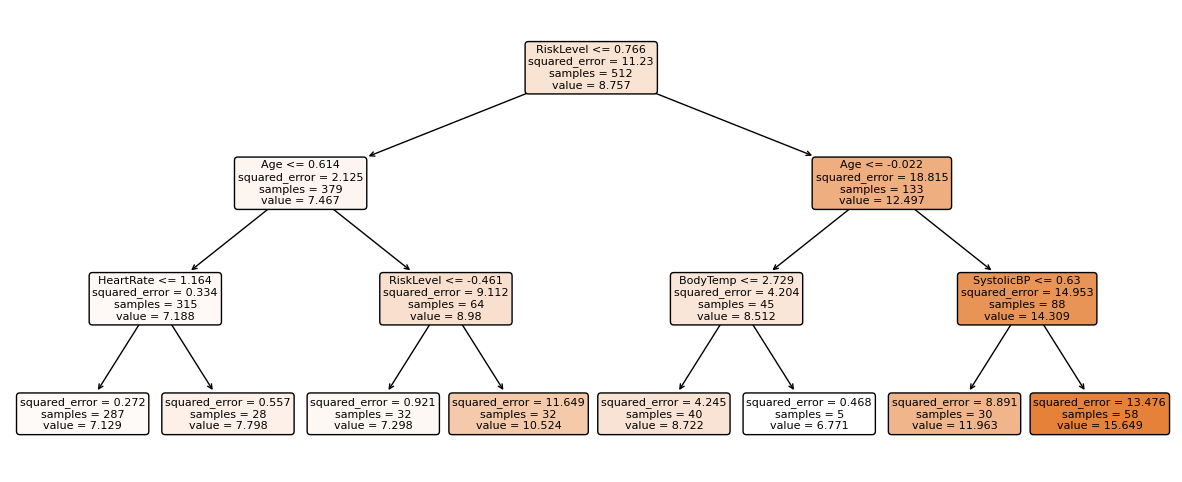Notice that the regression tree already has a value assigned to the data that falls on each node. Those are the values that will be averaged when combining the 20 trees. Folllowing what we have done with classification, you can also plot feature importances to see what variables the regression model is taking more into consideration when calculating values.

It is time to proceed to the last and final step when solving a machine learning problem and evaluate the performance of the algorithm!

##### Evaluating a RandomForestRegressor

For regression problems the metrics used to evaluate an algorithm are mean absolute error (MAE), mean squared error (MSE), and root mean squared error (RMSE).

1. Mean Absolute Error (MAE): when we subtract the predicted values from the actual values, obtaining the errors, sum the absolute values of those errors and get their mean. This metric gives a notion of the overall error for each prediction of the model, the smaller (closer to 0) the better.

$$mae = (\frac{1}{n})\sum_{i=1}^{n}\left | Actual – Predicted \right |$$Note: You may also encounter the y and ŷ notation in the equations. The y refers to the actual values and the ŷ to the predicted values.

1. Mean Squared Error (MSE): it is similar to the MAE metric, but it squares the absolute values of the errors. Also, as with MAE, the smaller, or closer to 0, the better. The MSE value is squared so as to make large errors even larger. One thing to pay close attention to, it that it is usually a hard metric to interpret due to the size of its values and of the fact that they aren’t in the same scale of the data.

$$mse = \sum_{i=1}^{D}(Actual – Predicted)^2$$

1. Root Mean Squared Error (RMSE): tries to solve the interpretation problem raised with the MSE by getting the square root of its final value, so as to scale it back to the same units of the data. It is easier to interpret and good when we need to display or show the actual value of the data with the error. It shows how much the data may vary, so, if we have an RMSE of 4.35, our model can make an error either because it added 4.35 to the actual value, or needed 4.35 to get to the actual value. The closer to 0, the better as well.

$$rmse = \sqrt{ \sum_{i=1}^{D}(Actual – Predicted)^2}$$

We can use any of those three metrics to compare models (if we need to choose one). We can also compare the same regression model with different argument values or with different data and then consider the evaluation metrics. This is known as hyperparameter tuning – tuning the hyperparameters that influence a learning algorithm and observing the results.

When choosing between models, the ones with the smallest errors, usually perform better. When monitoring models, if the metrics got worse, then a previous version of the model was better, or there was some significant alteration in the data for the model to perform worse than it was performing.

You can the following code to find these values:

from sklearn.metrics import mean_absolute_error, mean_squared_error print('Mean Absolute Error:', mean_absolute_error(y_test, y_pred))
print('Mean Squared Error:', mean_squared_error(y_test, y_pred))
print('Root Mean Squared Error:', np.sqrt(mean_squared_error(y_test, y_pred)))


The output should be:

Mean Absolute Error: 1.127893702896059
Mean Squared Error: 3.0802988503933326
Root Mean Squared Error: 1.755078018320933


With 20 trees, the root mean squared error is 1.75 which is low, but even so – by raising the number of trees and experimenting with the other parameters, this error could probably get even smaller.

### Advantages of using Random Forest

As with any algorithm, there are advantages and disadvantages to using it. In the next two sections we’ll take a look at the pros and cons of using random forest for classification and regression.

1. The random forest algorithm is not biased, since, there are multiple trees and each tree is trained on a random subset of data. Basically, the random forest algorithm relies on the power of “the crowd”; therefore the overall degree of bias of the algorithm is reduced.
2. This algorithm is very stable. Even if a new data point is introduced in the dataset the overall algorithm is not affected much since new data may impact one tree, but it is very hard for it to impact all the trees.
3. The random forest algorithm works well when you have both categorical and numerical features.
4. The random forest algorithm also works well when data has missing values or it has not been scaled.

### Disadvantages of using Random Forest

1. The main disadvantage of random forests lies in their complexity. They require much more computational resources, owing to the large number of decision trees joined together, when training large ensembles. Though – with modern hardware, training even a large random forest doesn’t take a lot of time.

### Going Further – Hand-Held End-To-End Project

Your inquisitive nature makes you want to go further? We recommend checking out our Guided Project: “Hands-On House Price Prediction – Machine Learning in Python”.In this guided project – you’ll learn how to build powerful traditional machine learning models as well as deep learning models, utilize Ensemble Learning and traing meta-learners to predict house prices from a bag of Scikit-Learn and Keras models.

Using Keras, the deep learning API built on top of Tensorflow, we’ll experiment with architectures, build an ensemble of stacked models and train a meta-learner neural network (level-1 model) to figure out the pricing of a house.

Deep learning is amazing – but before resorting to it, it’s advised to also attempt solving the problem with simpler techniques, such as with shallow learning algorithms. Our baseline performance will be based on a Random Forest Regression algorithm. Additionally – we’ll explore creating ensembles of models through Scikit-Learn via techniques such as bagging and voting.

This is an end-to-end project, and like all Machine Learning projects, we’ll start out with – with Exploratory Data Analysis, followed by Data Preprocessing and finally Building Shallow and Deep Learning Models to fit the data we’ve explored and cleaned previously.

Source: https://stackabuse.com/random-forest-algorithm-with-python-and-scikit-learn/